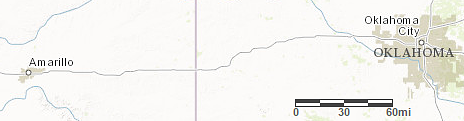# Lesson 8Scale Drawings and Maps

Let’s use scale drawings to solve problems.

### Learning Targets:

• I can use a map and its scale to solve problems about traveling.

## 8.1A Train and a Car

Two cities are 243 miles apart.

• It takes a train 4 hours to travel between the two cities at a constant speed.

• A car travels between the two cities at a constant speed of 65 miles per hour.

Which is traveling faster, the car or the train? Be prepared to explain your reasoning.

## 8.2Driving on I-90

1. A driver is traveling at a constant speed on Interstate 90 outside of Chicago. If she traveled from Point A to Point B in 8 minutes, did she obey the speed limit of 55 miles per hour? Explain your reasoning.
2. A traffic helicopter flew directly from Point A to Point B in 8 minutes. Did the helicopter travel faster or slower than the driver? Explain or show your reasoning.

Use the Distance tool

to measure the shortest distance between two points.

## 8.3Biking through Kansas

A cyclist rides at a constant speed of 15 miles per hour. At this speed, about how long would it take the cyclist to ride from Garden City to Dodge City, Kansas?“Map of Kansas” by United States Census Bureau via American Fact Finder. Public Domain.

### Are you ready for more?

Jada finds a map that says, “Note: This map is not to scale.” What do you think this means? Why is this information important?

## Lesson 8 Summary

Maps with scales are useful for making calculations involving speed, time, and distance. Here is a map of part of Alabama.

Suppose it takes a car 1 hour and 30 minutes to travel at constant speed from Birmingham to Montgomery. How fast is the car traveling?

To make an estimate, we need to know about how far it is from Birmingham to Montgomery. The scale of the map represents 20 miles, so we can estimate the distance between these cities is about 90 miles.

Since 90 miles in 1.5 hours is the same speed as 180 miles in 3 hours, the car is traveling about 60 miles per hour.

Suppose a car is traveling at a constant speed of 60 miles per hour from Montgomery to Centreville. How long will it take the car to make the trip? Using the scale, we can estimate that it is about 70 miles. Since 60 miles per hour is the same as 1 mile per minute, it will take the car about 70 minutes (or 1 hour and 10 minutes) to make this trip.

## Lesson 8 Practice Problems

1. Here is a map that shows parts of Texas and Oklahoma.“Map of Texas and Oklahoma” by United States Census Bureau via American Fact Finder. Public Domain.
1. About how far is it from Amarillo to Oklahoma City? Explain your reasoning.

2. Driving at a constant speed of 70 miles per hour, will it be possible to make this trip in 3 hours? Explain how you know.

2. A local park is in the shape of a square. A map of the local park is made with the scale 1 inch to 200 feet.

1. If the park is shown as a square on the map, each side of which is one foot long, how long is each side of the square park?
2. If a straight path in the park is 900 feet long, how long would the path be when represented on the map?